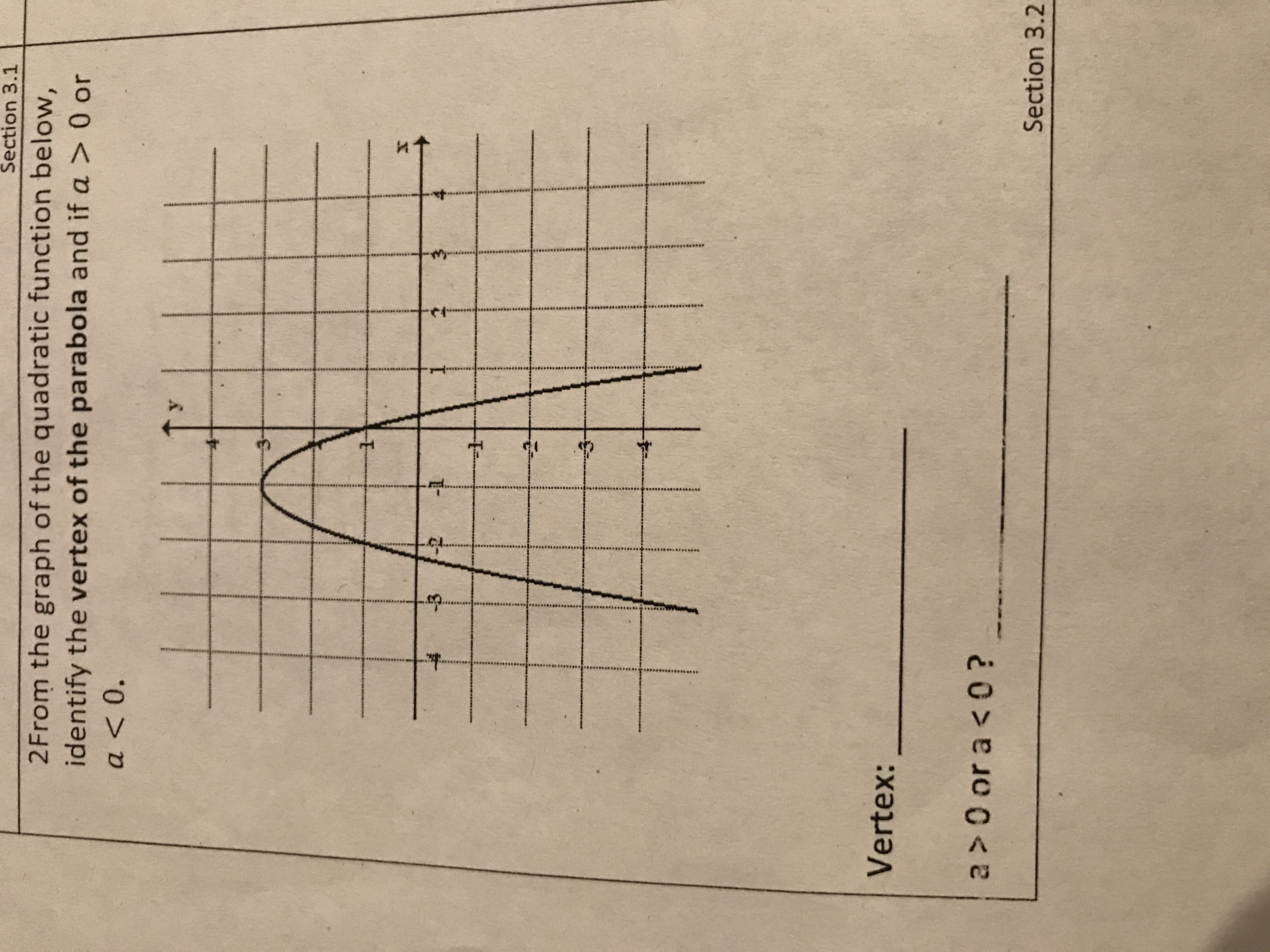# Section 3.12From the graph of the quadratic function below,identify the vertex of the parabola and if a > 0 ora 0 or a < 0?Section 3.2

Question
1 viewshelp_outlineImage TranscriptioncloseSection 3.1 2From the graph of the quadratic function below, identify the vertex of the parabola and if a > 0 or a <0. 1 Vertex: a > 0 or a < 0? Section 3.2 fullscreen
check_circle

Step 1

Since , parabola is downward parabola, so its equation is (x-h)^2=4a(y-k), where a is negative.

Step 2

Vertex of parabola is a point where the parabola crosses its axis of symmetry, that is...

### Want to see the full answer?

See Solution

#### Want to see this answer and more?

Solutions are written by subject experts who are available 24/7. Questions are typically answered within 1 hour.*

See Solution
*Response times may vary by subject and question.
Tagged in

### Other